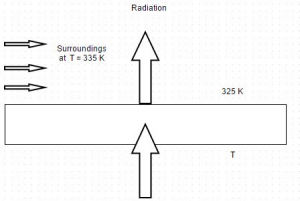# E-PolyLearning

 1. The diagram shows heat conduction through a plane wall. The surface temperature is 475 K and it radiates heat to the surroundings at 335 K. If thermal conductivity of the material is 12.5 W/m degree, find the temperature gradient. Let convective coefficient be 80 W/m² degree and radiation factor is 0.9a. – 1052.4 degree celsius b. – 2052.4 degree celsius c. – 3052.4 degree celsius d. – 4052.4 degree celsius

 2. From the concept of kinetic theory, mean travel velocity of the gas molecules is prescribed by the relation (Where, G = Universal gas constant M = Molecular weight of the gas T = Absolute temperature) a. V = (3M/GT)⅟₂ b. V = (3GT/M)⅟₂ c. V = (6T/GM)⅟₂ d. V = (3GT/2M)⅟₂